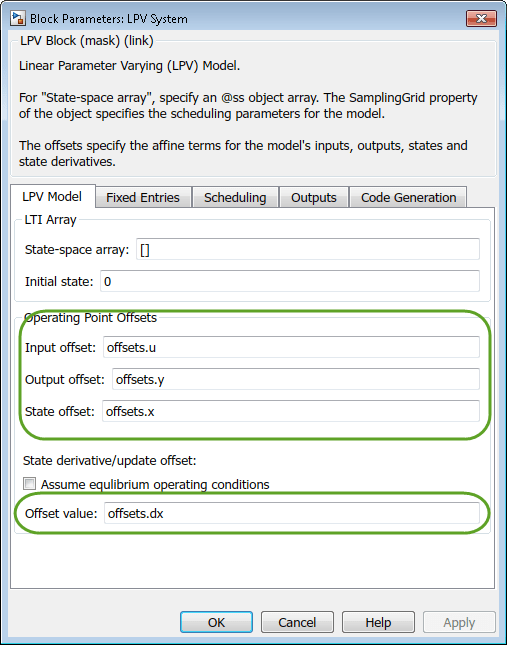Main Content

# getOffsetsForLPV

Extract LPV offsets from linearization results

## Syntax

``offsets = getOffsetsForLPV(info)``

## Description

example

````offsets = getOffsetsForLPV(info)` extracts linearization offsets from `info` and converts them to the array format supported by the LPV System block.```

## Examples

collapse all

Open the Simulink model.

```model = 'watertank'; open_system(model) ```Specify linearization I/Os.

```io(1) = linio('watertank/Desired Water Level',1,'input'); io(2) = linio('watertank/Water-Tank System',1,'output'); ```

Vary plant parameters `A` and `b`, and create a 3-by-4 parameter grid.

```[A_grid,b_grid] = ndgrid(linspace(0.9*A,1.1*A,3),linspace(0.9*b,1.1*b,4)); params(1).Name = 'A'; params(1).Value = A_grid; params(2).Name = 'b'; params(2).Value = b_grid; ```

Create a linearization option set, setting the `StoreOffsets` option to `true`.

```opt = linearizeOptions('StoreOffsets',true); ```

Linearize the model using the specified parameter grid, and return the linearization offsets in the `info` structure.

```[sys,op,info] = linearize('watertank',io,params,opt); ```

Extract the linearization offsets.

```offsets = getOffsetsForLPV(info) ```
```offsets = struct with fields: x: [2x1x3x4 double] y: [1x1x3x4 double] u: [1x1x3x4 double] dx: [2x1x3x4 double] ```

To configure an LPV System block, use the fields from `offsets` directly.## Input Arguments

collapse all

Linearization information returned by exact linearization commands, specified as a structure. This structure has an `Offsets` field that contains an N1-by-`...`-by-Nm array of structures, where N1 to Nm are the dimensions of the operating point array or parameter grid used for linearization. Each structure in `info.Offsets` contains offset information that corresponds to a specific operating point.

You can store and obtain linearization offsets when you linearize your model using one of the following commands:

For example:

```opt = linearizeOptions('StoreOffsets',true); [sys,op,info] = linearize(mdl,io,params,opt);```

You can then extract the offset information using `getOffsetsForLPV`.

`offsets = getOffsetsForLPV(info);`

## Output Arguments

collapse all

Linearization offsets corresponding to the operating points at which the model was linearized, returned as a structure with the following fields:

FieldDescription
`x`State offsets used for linearization, returned as an nx-by-1-by-N1-by-`...`-by-Nm array, where nx is the number of states in the linearized system.
`y`Output offsets used for linearization, returned as an ny-by-1-by-N1-by-`...`-by-Nm array, where ny is the number of outputs in the linearized system.
`u`Input offsets used for linearization, returned as an nu-by-1-by-N1-by-`...`-by-Nm array, where nu is the number of inputs in the linearized system.
`dx`Derivative offsets for continuous time systems, or updated state values for discrete-time systems, returned as an nx-by-1-by-N1-by-`...`-by-Nm array.

For instance, suppose that your model has three inputs, two outputs, and four states. If you linearize your model using a 5-by-6 array of operating points, `offsets` contains arrays with the following dimensions:

• `offsets.x` — 4-by-1-by-5-by-6

• `offsets.y` — 2-by-1-by-5-by-6

• `offsets.u` — 3-by-1-by-5-by-6

• `offsets.dx` — 4-by-1-by-5-by-6

To configure an LPV System block, you can use the fields of `offsets` directly. For an example, see Approximating Nonlinear Behavior Using an Array of LTI Systems.

## See Also

### Topics

Introduced in R2016b

## SupportGet trial now